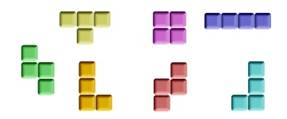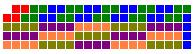# 第4届华为编程大赛决赛试题

=======================================================================

## 编程题（共1题，100分。请上机编写程序，按题目要求提交文件。测试用例不对考生公开，凡不满足提交要求导致不能运行或用例不通过，不予评分。）

### 1.俄罗斯方块之棋盘覆盖障碍说明：2 3 22 231、  用所提供的方块尽可能覆盖棋盘并输出结果；

2、  在（1）的基础上，棋盘上的空格越少越好。

C/C++：需要提交可执行文件和压缩打包的源代码工程

JAVA：需要提交压缩打包的整个编码工程目录

==============================================================

============================================================================（右下角也是完全一样）

 123456789101112131415161718192021222324252627282930313233343536373839404142434445464748495051525354555657585960616263646566676869707172737475767778798081828384858687888990919293949596979899100101102103104105106107108109110111112113114115116117118119120121122123124125126127128129130 #include #include #include #define TABLE_SIZE 21 // store the input int input = {0}; // store the hole answer char table[TABLE_SIZE * TABLE_SIZE]; bool isVertical = false; void Initialize(); void Output(FILE* f); void Construct(); int main(){     FILE* fw = fopen("testout.txt", "w");     FILE* fp = fopen("testin.txt", "r");     if (!fp){         printf("file not found!\n");         return 0;     }     // main loop     while (!feof(fp)){              fscanf(fp, "%d %d %d %d", &input, &input, &input, &input);         Initialize();         Construct();         Output(fw);     }     fclose(fp);     fclose(fw);     return 0; } void Initialize(){     int i, j, x, y;     memset(table, 'f', sizeof(table));     for (i = 0; i < 4; ++i){         if (input[i] < 1 || input[i] > TABLE_SIZE * TABLE_SIZE){             printf("input number error!\n");             exit(0);         }         --input[i]; // input pos start from 1... but I start from 0     }     //sort input     for (i = 1; i < 4; ++i){         if (input[i] < input[i - 1]){             int temp = input[i];             for (j = i - 1; j >= 0 && input[j] > temp; --j){                 input[j + 1] = input[j];             }             input[j + 1] = temp;         }     }     //rotate input to 3x2 if it is 2x3     if (input - input != 1){         isVertical = true;         for (i = 0; i < 4; ++i){             x = input[i] % TABLE_SIZE;             y = input[i] / TABLE_SIZE;             input[i] = x * TABLE_SIZE + TABLE_SIZE - 1 - y;         }         input ^= input;         input ^= input;         input ^= input;     }     } void Construct(){     int i, j, x, y;     x = input % TABLE_SIZE;     y = input / TABLE_SIZE;     if (input == 1 || input == TABLE_SIZE * TABLE_SIZE - 2 - TABLE_SIZE){ // in the corner (left-top or right-bottom)         if (input == 1){             table = '0';         }else{             table[TABLE_SIZE * TABLE_SIZE - 1] = '0';         }         for (i = 1; i < TABLE_SIZE; ++i){             table[i + y * TABLE_SIZE] = 'a';             table[i - 1 + (y + 1) * TABLE_SIZE] = 'a';         }     }else{         //fill it with L in a 4x3 tiny block (3x4 is also useful)         if (y != TABLE_SIZE - 2 && x != 1){             for (i = x - 2; i < x + 2; ++i){                 for (j = y; j < y + 3; ++j){                     table[i + j * TABLE_SIZE] = 'c';                 }             }         }else{             for (i = x - 1; i < x + 3; ++i){                 for (j = y - 1; j < y + 2; ++j){                     table[i + j * TABLE_SIZE] = 'c';                 }             }           }           //leave a hole anywhere         if (table == 'f'){             table = '0';         }else{             table[TABLE_SIZE - 1] = '0';            }           }     // fill the barrier     for (i = 0; i < 4; ++i){         table[input[i]] = '1';     } } void Output(FILE* fp){     int i, j;     for (i = 0; i < TABLE_SIZE; ++i){         for (j = 0; j < TABLE_SIZE; ++j){             if (isVertical){                 fprintf(fp, "%c ", table[TABLE_SIZE - 1 - i + j * TABLE_SIZE]);             }else{                 fprintf(fp, "%c ", table[i * TABLE_SIZE + j]);                          }         }         fprintf(fp, "\n");     }     fprintf(fp, "1\n"); }

### 12 条评论

1.匿名 说道：

2 3 22 23什么意思？

•suika 说道：

表示在第2、第3、第22、第23这4个位置有障碍，如果以21x21大小的棋盘左上角为1，先横着数再竖着数，则这4个位置正好构成图中所示的那个俄罗斯方块图案

2.匿名 说道：

顶一个！！！

3.匿名 说道：

棋盘共有441个格子，每个现状都是4格，441%4 = 1;剩下的空格数只可能是1,5,9,13.。。
直接暴力求解，每种障碍都能算出剩一个空格的布局。已经是最优的了

•suika 说道：

感谢提醒，但是直接暴搜好像很慢啊，110个图案填进去的组合数太多了。如果先缩小区域再暴搜，主要的障碍是对小区域内能达成最优（即剩1或0个格子）的证明。这个证明只能靠枚举么？

•匿名 说道：

有同学做了，对任何位置的障碍都能填充到只剩一个空格（本来至少会留一个空格 ）。先和你得做法一样对4的倍数的行（不包含障碍的行）用f填满，缩小范围。这时其实在下面用笔分析出了只剩一个空格的填充法，没有在程序里搜，程序里面只是一些赋值。。。只要做出一种障碍，另外一种障碍旋转得到第一种障碍，再解决就行了。。

4.匿名 说道：

我做出来的结果都是5，如果要求都是1的话，程序要更复杂，没时间写了。

5.ctzsm 说道：

其实我想知道有多少人是构造的。。。。。

而且其实构造的时候有个小trick，有一个情况下构造3*4（对于您这种情况，我的情况是4*3）的方块的时候，会把那个空白覆盖掉。我是写了一个check才发现的。没有太多时间看您的程序，不知道是否有相似的问题。

•suika 说道：

抱歉……稍微有些没看懂您的意思
文后那份代码里，在确定了构造4x3 / 3x4的矩形之后，其他地方就全用长条填充，再随意留一个空就可以了。只要先确定矩形位置，再确定留空的位置，就不会让空隙被覆盖了。

•ctzsm 说道：

我的填充方法跟你略有差异。

- -表示当时直接用excel画了一下，于是。。。我还在压缩包里加了个excel文件。。。

这题比前两届的简单多了，不知道华为最后怎么评判。。。

6.杨旭光 说道：

我边拿笔算边看题目，我想到的是将其障碍变成4x3的障碍，就如你所说的4x3的障碍。可是就没想到剩下的分配了...哈哈。自己也忍不住往下看你的做法，就没再想直接跳到你的算法中。

7.mtkfish 说道：

dsd